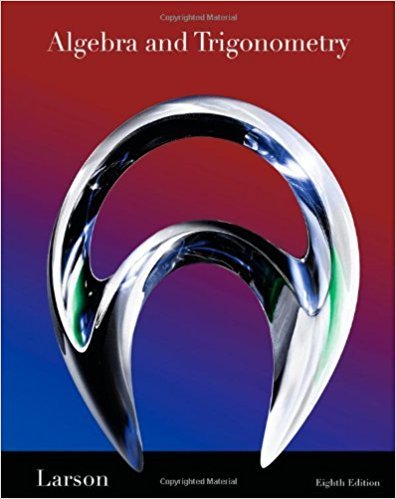×
×

# Solutions for Chapter 4: Rational Functions and Conics## Full solutions for Algebra and Trigonometry | 8th Edition

ISBN: 9781439048474Solutions for Chapter 4: Rational Functions and Conics

Solutions for Chapter 4
4 5 0 379 Reviews
16
0
##### ISBN: 9781439048474

Algebra and Trigonometry was written by and is associated to the ISBN: 9781439048474. This expansive textbook survival guide covers the following chapters and their solutions. Since 89 problems in chapter 4: Rational Functions and Conics have been answered, more than 47711 students have viewed full step-by-step solutions from this chapter. This textbook survival guide was created for the textbook: Algebra and Trigonometry, edition: 8. Chapter 4: Rational Functions and Conics includes 89 full step-by-step solutions.

Key Math Terms and definitions covered in this textbook
• Cholesky factorization

A = CTC = (L.J]))(L.J]))T for positive definite A.

• Circulant matrix C.

Constant diagonals wrap around as in cyclic shift S. Every circulant is Col + CIS + ... + Cn_lSn - l . Cx = convolution c * x. Eigenvectors in F.

• Diagonal matrix D.

dij = 0 if i #- j. Block-diagonal: zero outside square blocks Du.

• Diagonalization

A = S-1 AS. A = eigenvalue matrix and S = eigenvector matrix of A. A must have n independent eigenvectors to make S invertible. All Ak = SA k S-I.

• Elimination.

A sequence of row operations that reduces A to an upper triangular U or to the reduced form R = rref(A). Then A = LU with multipliers eO in L, or P A = L U with row exchanges in P, or E A = R with an invertible E.

• Gauss-Jordan method.

Invert A by row operations on [A I] to reach [I A-I].

• Hilbert matrix hilb(n).

Entries HU = 1/(i + j -1) = Jd X i- 1 xj-1dx. Positive definite but extremely small Amin and large condition number: H is ill-conditioned.

• Incidence matrix of a directed graph.

The m by n edge-node incidence matrix has a row for each edge (node i to node j), with entries -1 and 1 in columns i and j .

• Indefinite matrix.

A symmetric matrix with eigenvalues of both signs (+ and - ).

• Kirchhoff's Laws.

Current Law: net current (in minus out) is zero at each node. Voltage Law: Potential differences (voltage drops) add to zero around any closed loop.

• Krylov subspace Kj(A, b).

The subspace spanned by b, Ab, ... , Aj-Ib. Numerical methods approximate A -I b by x j with residual b - Ax j in this subspace. A good basis for K j requires only multiplication by A at each step.

• Lucas numbers

Ln = 2,J, 3, 4, ... satisfy Ln = L n- l +Ln- 2 = A1 +A~, with AI, A2 = (1 ± -/5)/2 from the Fibonacci matrix U~]' Compare Lo = 2 with Fo = O.

• Minimal polynomial of A.

The lowest degree polynomial with meA) = zero matrix. This is peA) = det(A - AI) if no eigenvalues are repeated; always meA) divides peA).

• Nullspace matrix N.

The columns of N are the n - r special solutions to As = O.

• Orthogonal matrix Q.

Square matrix with orthonormal columns, so QT = Q-l. Preserves length and angles, IIQxll = IIxll and (QX)T(Qy) = xTy. AlllAI = 1, with orthogonal eigenvectors. Examples: Rotation, reflection, permutation.

• Positive definite matrix A.

Symmetric matrix with positive eigenvalues and positive pivots. Definition: x T Ax > 0 unless x = O. Then A = LDLT with diag(D» O.

• Row picture of Ax = b.

Each equation gives a plane in Rn; the planes intersect at x.

• Saddle point of I(x}, ... ,xn ).

A point where the first derivatives of I are zero and the second derivative matrix (a2 II aXi ax j = Hessian matrix) is indefinite.

• Special solutions to As = O.

One free variable is Si = 1, other free variables = o.

• Standard basis for Rn.

Columns of n by n identity matrix (written i ,j ,k in R3).

×